# Use R to Easily Estimate Migration Matrices with RTransProb (Part 1)

This blog introduces my R package, RTransprob. The RTransprob package contains a set of functions used to automate commonly used methods to estimate migration matrices used in credit risk analysis. This includes methods for estimating migration and default rates based on the duration and cohort methods, bootstrapping default rates and forecasting/stress testing credit exposures migrations, via Econometrics and a couple of Machine Learning algorithms.

### Motivation

As the popularity of R continues to grow, individual developers and even entire departments have migrated from other competing platforms (Matlab, SAS, etc …).  R provides some advantages over each of these other development environments, in particular:

• R has a very active community. Users have contributed over 10,000 packages (as of July 6, 2017)
• R is FREE! Matlab, SAS, S-Plus, and others can cost thousands of dollars
• R provides syntax which is very familiar to developers versed in other languages. This observation is not to be underestimated.

However, one drawback of migrating to R is simply that not all models or code built on a legacy platform are easily ported (or translated) to R.  When programmers raise the conversion conversation (ha, say that 10 times), the expectation is that most (if not all) of the functionality that exists in the legacy platforms also exists in one form or another in R. Sometimes, no such luck.

While working as a consultant for a rather large financial institution, I ran into such a problem.  I was hired to redevelop several CCAR/DFAST credit stress models. CCAR and DFAST are two sets of regulatory exercises that are mandated to larger financial institutions, and were developed as a result of the passing of the Dodd-Frank Consumer Protection Act. Specifically, I was tasked to deliver 2nd generation Probability of Default models. The analysts employed by my client were Matlab and SAS users. As a result, all of their previous models were built using Matlab and SAS.

### Credit Migration Matrices

As part of the CCAR/DFAST annual stress testing analysis, my client (as others banks of similar size) was required to forecast its expected losses applied against the Wholesale loan portfolio over a nine quarter forecast interval, under a suite of macroeconomic scenarios prescribed by the Federal Reserve Board. These hypothetical forecasts are run for each line of business within the bank, and are designed to evaluate the institution capital adequacy levels under varying degrees of economic severity.

In finance, a credit rating framework provides a method to standardize information about credit quality, by ranking the borrowers (and loans) according to their credit-worthiness.  While the ranking is in itself useful, institutions are also interested in understanding the behaviors of the rating categories over specified lengths of time. For example: what is the probability of a borrower rated migrating to a better credit rating? what is the probability of a borrower migrating to a worse credit rating? what is the probability of a borrower defaulting on its financial obligations? Quantitative answers to these questions serve as inputs into many credit risk management models (including credit portfolio loss models) in the form of migration matrices.

The matrices contain credit migration probabilities, which characterize historical changes in the financial strength of borrowers, which are typically firms. When observed together, these migration probabilities can describe the trajectory of an entity’s credit path in a migration matrix.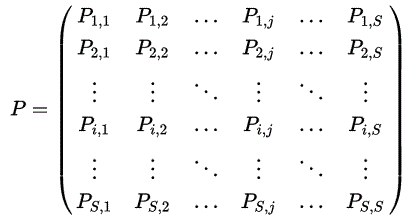Now, back to the client. The legacy models at the firm i was consulting with, made use of a very useful set of functions available in the Matlab Financial ToolboxTM, which easily enables analysts to incorporate credit migrations into their models. The equivalent set of functions or package needed to mimic the Matlab migration functionality did not exist in R.  Out of necessity, I developed a migration matrix library. This blog introduces the resulting R package RTransprob that was created and which is currently available on the R package warehouse CRAN.

While more sophisticated methods exist for deriving migration matrices used for estimating transition probabilities, the two most common methods are using the cohort and duration (also known as hazard rate or intensity) approaches.

Simply understood, the cohort method captures rating transitions at the beginning and the end of an observation period, i.e. in each cohort. In an example with a portfolio containing loans consisting of only 3 states A, B, and C the cohort approach counts the transitions from A → B, A → C,  B → A, B → C, etc… along with the number of loans that remained in each state and calculates each transition probability by comparison to the beginning counts in each state. On the other hand, the duration method concentrates on transition intensities, which essentially describe how long a borrower is expected to stay in each state during the observation period.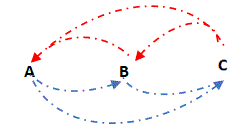### Estimate Transition Probabilities

We will demonstrate basic usage of the RTransprob package using several examples below. It should be noted however that this is part 1 of a 2 part series. Part 2 will focus on the use of the package to forecasting/stress testing credit exposures migrations.

```library("RTransProb")
```

Next review the sample data set (`'dataTM'` ) included with the package. The `'dataTM'` file contains the following sample credit ratings data.

```head(dataTM)
ID     Date    Rating Num_Ratings
1 1   5/30/2000   G       7
2 1  12/31/2000   F       6
3 2   5/21/2003   F       6
4 3  12/30/1999   E       5
5 3  10/30/2000   F       6
6 3  12/30/2001   E       5
```

The sample data is formatted as a dataframe with four columns. Each row contains an id (ID), a date (Date), an alphanumeric credit rating (Rating), and a numeric credit rating (Num_Ratings). The assigned credit rating corresponds to the associated ID on the associated date. All information corresponding to the same ID must be stored in contiguous rows. In this example, ID and Rating are stored as an ‘int’, Date and Num_Ratings are both stored as a ‘factor’. Also note that the data is sorted on ID and Date.

The main function in the package is TransitionProb(). This function takes the following arguements:

 dataTM a table containing historical credit ratings data (i.e., credit migration data). A dataframe of size nRecords x 4 where each row contains an ID (column 1), a date (column 2), a credit rating (column 3), and a numeric credit rating (column 4); The credit rating is the rating assigned to the corresponding ID on the corresponding date. startDate start date of the estimation time window, in string or numeric format. If an ‘startDate’ is not specified, the default start date is the earliest date in ’dataTM’. endDate end date of the estimation time window, in string or numeric format. If an ‘endDate’ is not specified, the default end date is the latest date in ’dataTM’. The end date cannot be a date before the start date. method estimation algorithm, in string format. Valid values are ’duration’, ’cohort’ or ‘tnh’. snapshots integer indicating the number of credit-rating snapshots per year to be considered for the estimation. Valid values are 1, 4, or 12. The default value is 1, i.e., one snapshot per year. This parameter is only used in the ’cohort’ method. interval the length of the transition interval under consideration, in years. The default value is 1, i.e., 1-year transition probabilities are estimated.

Cohort Method vs Duration Method

The cohort method takes snapshots of credit ratings at regularly spaced points in time over the specified time interval of interest (eg. 1 snapshot over the time window 2000-01-01 through 2001-01-01 would results in a single 1 year interval; 2 snapshots over the time window 2000-01-01 through 2001-01-01, would results in a 2 six month intervals; 4 snapshots over the time window 2000-01-01 through 2001-01-01, would results in quarterly intervals, etc. ).

The cohort method uses all of the ratings available at the beginning of the interval as the base reference. Any rating changes which occur within the interval are overlooked and only the initial and final ratings are considered in the estimates.

The duration method uses all of the available ratings change information the by considering the exact dates on which the credit rating migrations occur. Since the timing and sequence of the ratings changes As a result the snapshots

Example 1:
Using the cohort method, when start date (“startDate”) and end date (“endDate”) are not specified, the entire data set is used and the package performs TTC calculations. When snapshots and interval are not specified the defaults are 1 (i.e.  this results in a yearly snapshots of the credit ratings and 1-year transition probabilities)

```snapshots = 0
interval  = 0
startDate = 0
endDate   = 0
Example1  = TransitionProb(dataTM,startDate,endDate,'cohort', snapshots, interval)
```

to display the migration matrix output enter `> Example1\$transMat`.The ‘sampleTotals’  list is an optional output from the TransitionProb() function. As displayed in the tables below, when the ‘cohort’ method is used, the ‘sampleTotals’ list contains summary information on (1) the total number of borrowers in each credit rating bucket (sampleTotals\$totalsVec) and (2) number of transitions out of each credit rating (sampleTotals\$totalsMat).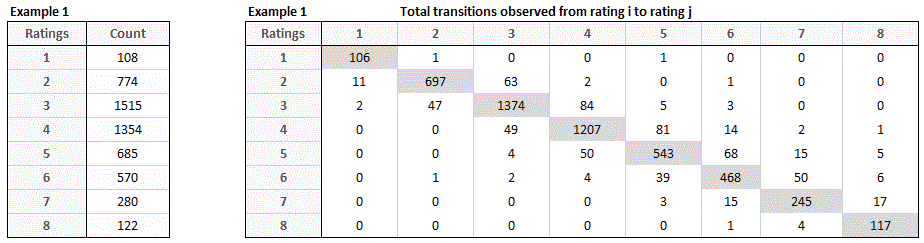Example 2:
Using the duration method,  when the window of interest is specified as a 2-year period from the end of 2000 to the end of 2002. The snapshots and interval are not specified. When snapshots and interval are not specified the defaults are 1 (i.e.  this results in a yearly snapshots of the credit ratings and 1-year transition probabilities)

```snapshots = 0
interval  = 0
startDate = "2000-01-01"
endDate   = "2002-01-01"
Example2  = TransitionProb(dataTM,startDate, endDate,'duration', snapshots, interval)
```The ‘sampleTotals’  list is an optional output from the TransitionProb() function. When the  ‘duration’ method is used the ‘sampleTotals’ list contains summary information on (1)  the total time spent on each rating (sampleTotals\$totalsVec),  (2) the number of transitions out of each rating (sampleTotals\$totalsMat),and (3) a generator matrix (sampleTotals\$genMat).Example 3:
Using the cohort method,  the time window of interest is the 3-year period from the beginning of 2000 to the beginning of 2003. We want to estimate 1-year transition probabilities using cohort method. When snapshots and interval are not specified the defaults are 1 (i.e.  this results in a yearly snapshots of the credit ratings and 1-year transition probabilities)

```snapshots = 0
interval  = 0
startDate = "2000-01-01"
endDate   = "2003-01-01"
Example3  = TransitionProb(dataTM,startDate, endDate,'cohort', snapshots, interval)
```Example 4:
assume that the time window of interest is the 5-year period from the beginning of 2000 to the beginning of 2005. We want to estimate 1-year transition probabilities using quarterly snapshots using cohort method.

```snapshots = 4 #This uses quarterly transition matrices
interval  = 1 #This gives a 1 year transition matrix
startDate = "2000-01-01"
endDate   = "2005-01-01"
Example4  = TransitionProb(dataTM,startDate, endDate,'cohort', snapshots, interval)
```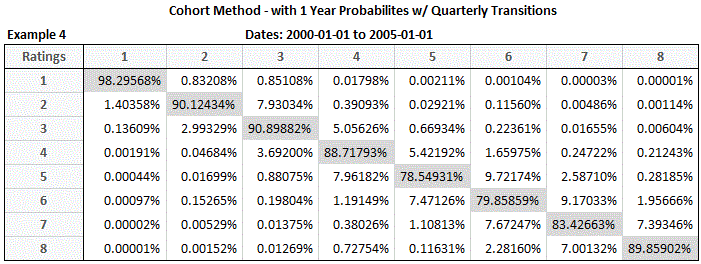Example 5:
assume that the time window of interest is the 5-year period from the beginning of 2000 to the beginning of 2005. We want to estimate a 2-year transition probabilities using quarterly snapshots using cohort method

```snapshots = 4 #This uses quarterly transition matrices
interval  = 2 #This gives a 2 years transition matrix
startDate = "2000-01-01"
endDate   = "2005-01-01"
Example5  = TransitionProb(dataTM,startDate, endDate,'cohort', snapshots, interval)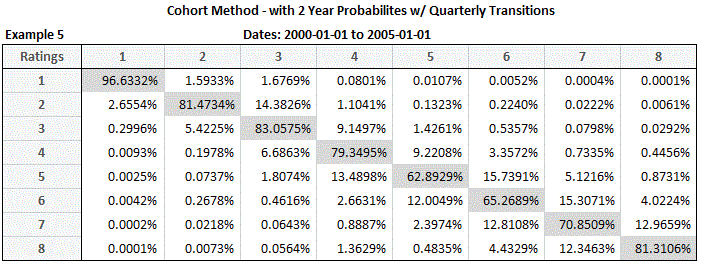Example 6:
assume that the time window of interest is the 2-year period from the beginning of 2000 to the beginning of 2002. We want to estimate 2-year transition probabilities using quarterly snapshots using duration method.
snapshots  = 4 #This uses quarterly transition matrices
interval   = 2 #This gives a 2 year transition matrix
startDate  = "2000-01-01"
endDate    = "2002-01-01"
Example6   = TransitionProb(dataTM,startDate, endDate,'duration', snapshots, interval)Example 7:
assume that the time window of interest is the 5-year period from the beginning of 2000 to the beginning of 2005. We want to estimate 1-year transition probabilities using monthly snapshots using cohort method
snapshots = 12 #This uses monthly transition matrices
interval  = 1 #This gives a 1 year transition matrix
startDate = "2000-01-01"
endDate   = "2005-01-01"
Example7  = TransitionProb(dataTM,startDate, endDate,'cohort', snapshots, interval)Example 8:
assume that the time window of interest is the 5-year period from the beginning of 2000 to the beginning of 2005. We want to estimate 1-year transition probabilities using annual snapshots using cohort method.
snapshots = 1 #This uses annual transition matrices
interval  = 1 #This gives a 1 year transition matrix
startDate = "2000-01-01"
endDate   = "2005-01-01"
Example8  = TransitionProb(dataTM,startDate, endDate,'cohort', snapshots, interval)

Through-the-Cycle Estimation and Bootstrapped Confidence Interval
Point-in-Time vs Through-the-Cycle Estimation:
There are two different philosophies most often used to describe the behaviour of the credit rating systems; Point-in-Time (PIT) and Through-the-Cycle (TTC).  PIT credit transition matrices, are estimated using a narrow estimation window,  thus describing rating systems which adhere to the business cycle and change over time to reflect the changes in the business cycle. Conversely, the TTC philosophy is to estimate transition matrices over a large enough time frame to dampen the effects of economical conditions.  TTC transition rate are thus presumed to be cycle neutral.
The package RTransProb, can be used to generate easily Point-in-Time transition matrices which are then used to estimate Through-the-Cycle transition matrices and finally construct bootstrapped confidence intervals.
Below we illustrate how to :
1) Generate cohort Point-In-Time transition matrices using the getPIT() function.
2) Using the output from getPIT() in step 1, we estimate a through-the-cycle transition matrix using the cohort.TTC() function.
3) Finally, we estimate bootstrapped confidence intervals using the cohort.CI() function.
cohort.ttc() provides several averaging methods used to 'average' the transition matrices as a result the function.  Valid averaging methods are:

SAT
{Scaled Average Transitions} - compute a TTC transition matrix by first scaling and weighting the counts (initial counts and transition counts) then calculate periodic transition matrices, and finally averaging over all available periods. e.g., average January matrices, then February matrices or average Q1, then Q2 ...then obtain the average of the transition matrices

SAPT
{Scaled Average Periodic Transitions} - compute a TTC transition matrix by weighting the periodic transition percentages (calculate the period transition matrices then weigh the percentages, and finally averaging over all available periods. e.g., average January matrices, then February matrices or average Q1, then Q2 ...then obtain the average of the transition matrices

USAT
{Unscaled Average Transitions} - compute a TTC transition matrix by first obtaining unscaled periodic transition matrices then averaging over all available periods.

ATMP
{Average Transition Matrices By Period} - returns the weighted the periodic transition percentages (calculate the periodic transition matrices then weigh the percentages.

ATP
{Average Transitions By Period} - returns the scaled periodic transitions.

ACP
{Average Count By Period} - returns the scaled periodic initial counts.

#Generate Point-in-Time transition matrices using the  getPIT() function
startDate  = "2003-01-01"
endDate    = "2005-01-01"
snapshots  = 4 #This uses quarterly snapshots
interval   = 0.25 #This gives quarterly transition matrix
ExamplePIT = getPIT(dataTM,startDate, endDate,'cohort', snapshots, interval)

#use the output from 'ExamplePIT' as parameters into cohort.ttc(),
#to generate the Through-the-Cycle transition matrices.
lstInit = Example\$lstInitVec[lapply(Example\$lstInitVec,length)&amp;gt;0]
lstCnt  = Example\$lstCntMat[lapply(Example\$lstCntMat,length)&amp;gt;0]
ExampleTTC = cohort.TTC(lstCnt,lstInit)

#use \$ATMP from the cohort.TTC() as the input into the cohort.CI() function
transMatrix = ExampleTTC\$ATMP
initCount = ExampleTTC\$ACP[][,1]
sim = 1000
tolerance_Cohort = cohort.CI(transMatrix,initCount,sim)Below we illustrate how to :
1) Generate cohort Point-In-Time transition matrices using the getPIT() function.
2) Using the output from getPIT() in step 1, we estimate a through-the-cycle transition matrix using the duration.TTC() function.
3) Finally, we estimate bootstrapped confidence intervals using the duration.CI() function.
The output from the duration.ttc()

CLW
{Count Level Weighting} - Construct TTC transition matrix from scaled and weighted counts data (transitions and initial state count)

PTMLW
{Periodic Transition Matrix Level Weighting} - Construct TTC transition matrix using the average of all of the weighted periodic transition matrices(Scaling is performed at the periodic transition matrix level).

PGMLW
{Unscaled and UnWeighted Periodic Transition Matrices} - Construction of unscaled and unweighted periodic transition matrices from unscaled and unweighted periodic generator matrices.

UUPTM
{Unscaled and UnWeighted Periodic Transition Matrices} - Construction of unscaled and unweighted periodic transition matrices from unscaled and unweighted periodic generator matrices.

WGM
{Weighted Generator Matrix} - Average periodic generator matrices.

SWT
{Scaled and Weighted Transitions} - scaled weighted transitions

SWFY
{Scaled and Weighted Firm Years} - scaled weighted firm years

#Generate Point-in-Time transition matrices using the  getPIT() function
startDate = "2003-01-01"
endDate   = "2005-01-01"
snapshots  = 4 #This uses quarterly snapshots
interval   = 0 #This gives quarterly transition matrix
ExamplePIT = getPIT(dataTM,startDate, endDate,'duration', snapshots, interval)

#use the output from 'ExamplePIT' as parameters into duration.ttc(),
#to generate the Through-the-Cycle transition matrices.
lstInit = Example1\$lstInitVec[lapply(Example1\$lstInitVec,length)&amp;amp;amp;gt;0]
lstCnt  = Example1\$lstCntMat[lapply(Example1\$lstCntMat,length)&amp;amp;amp;gt;0]
ExampleTTC1 = duration.TTC(Example1\$lstCntMat,Example1\$lstInitVec)

portWgts = ExampleTTC1\$SWFY[,1]
nHorizon = length(ExampleTTC1\$UUPTM[])
sim = 100
tolerance_Duration = duration.CI(ExampleTTC1\$CLW,portWgts,nHorizon,sim)

transtype = "AAT" #("APTA", "GAPTA","RDAA","AAT") #averaging method
transMatTest = cohort.TTC(lstCnt,lstInit,snapshots,transtype)
portWeights = c(5,10,15,20,20,20,15,5)
nPeriods = 4
nStates = 8
sim = 1000
tolerance_Cohort = cohort.CI(transMatTest\$averageTransMatByPeriod,portWeights,snapshots,nStates,sim)

That’s all for now. In Part 2, we’ll demonstrate how to use RTransProb to condition the transition matrices based on economic factors.

## 5 thoughts on “Use R to Easily Estimate Migration Matrices with RTransProb (Part 1)”

1.Dominic Joseph says:

Hi, great blog. May I know why the output of bootstrap confidence interval matrix doesn’t show any intervals?

2.Dominic Joseph says:

Hi nice blog btw

3. […] The matrices contain credit migration probabilities, which characterize historical changes in the financial strength of borrowers. When observed together, these migration probabilities can describe the trajectory of an entity’s credit path in a migration matrix. (For more information on estimating migration matrices please see my article “Use R to Easily Estimate Migration Matrices with RTransProb (Part 1)”.) […]

4.Alex says:

I receive this error when I subset data per one ID

error: SpMat::operator(): out of bounds
Error in getidTotCntCohortRCPP(nIDs, numDate, numericalRating, as.numeric(StartPos[, :
SpMat::operator(): out of bounds

On the other hand , it works for the whole set of data

5.Gosha says:

Hi! Thanks for the article, it was very informative 🙂
I’m currently using R version 3.5.1, and it seems that RTransProb isn’t available under this version.
Is there any updated version of this package or could you advise on some other packages I can use to build transition matrices?

Thanks a lot!

This site uses Akismet to reduce spam. Learn how your comment data is processed.